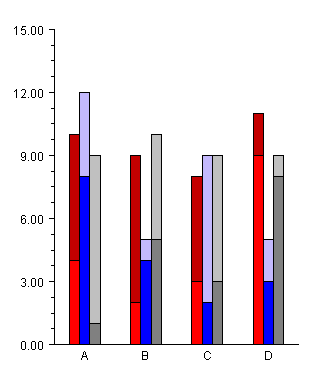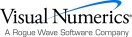## Stacked Grouped Bar Chart

The most general form of the bar chart is a stacked, grouped bar chart.

The data argument to the constructor for a stacked, grouped bar chart is an nStacks by nGroups by nItems array of double. In this example there are two stacks in three groups each containing four items. All of the stacks must contain the same number of groups and all of the groups must contain the same number of items.

The GetBarSet(int,int) method returns a BarSet object that is a collection of the BarItems that make up a given stack/group. Here within each group the stacks are set to shades of the same color.

A stacked bar chart, without groups, can be constructed as a stacked-grouped bar chart with one groupusing Imsl.Chart2D;

using System.Drawing;

public class SampleBarGroupStack : FrameChart {

public SampleBarGroupStack() {

Chart chart = this.Chart;

AxisXY axis = new AxisXY(chart);

// y is a 2 by 3 by 4 array

double[][][] y = new double[][] {

new double[] {

new double[] {4, 2, 3, 9},

new double[] {8, 4, 2, 3},

new double[] {1, 5, 3, 8}},

new double[] {

new double[] {6, 7, 5, 2},

new double[] {4, 1, 7, 2},

new double[] {8, 5, 6, 1}}};

Bar bar = new Bar(axis, y);

bar.BarType = Bar.BAR_TYPE_VERTICAL;

bar.SetLabels(new string[] {"A","B","C","D"});

// group 0 - shades of red

bar.GetBarSet(0,0).FillColor = Color.Red;

bar.GetBarSet(1,0).FillColor = Color.DarkRed;

// group 1 - shades of blue

bar.GetBarSet(0,1).FillColor = Color.Blue;

bar.GetBarSet(1,1).FillColor = Color.LightBlue;

// group 2 - shades of gray

bar.GetBarSet(0,2).FillColor = Color.Gray;

bar.GetBarSet(1,2).FillColor = Color.LightGray;

}

public static void Main(string[] argv) {

System.Windows.Forms.Application.Run(

new SampleBarGroupStack());

}

}Visual Numerics - Developers of IMSL and PV-WAVEhttp://www.vni.com/PHONE: 713.784.3131FAX:713.781.9260